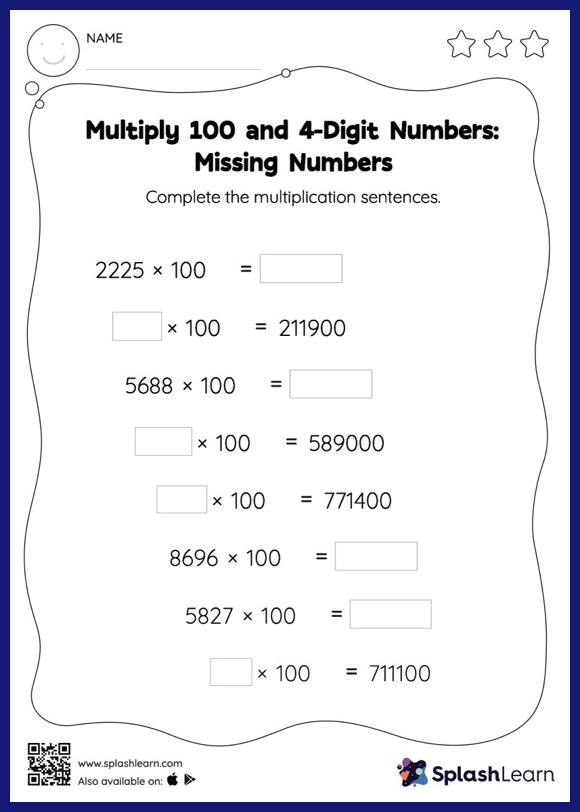# Multiply 100 and 4-Digit Numbers: Missing Numbers Worksheet

Home > Multiply 100 and 4-Digit Numbers: Missing NumbersStudents demonstrate their problem-solving ability as they work on this multiply 100 and 4-digit numbers worksheet. When asked to multiply numbers by 10, 100, or their multiples, students use the pattern of zeros. They use the same idea to find the missing number in multiply 100 and 4-digit numbers worksheet.